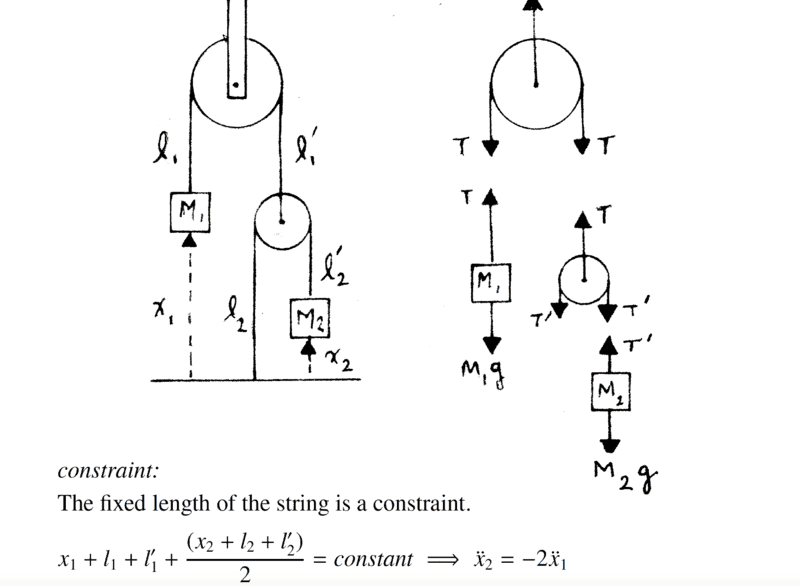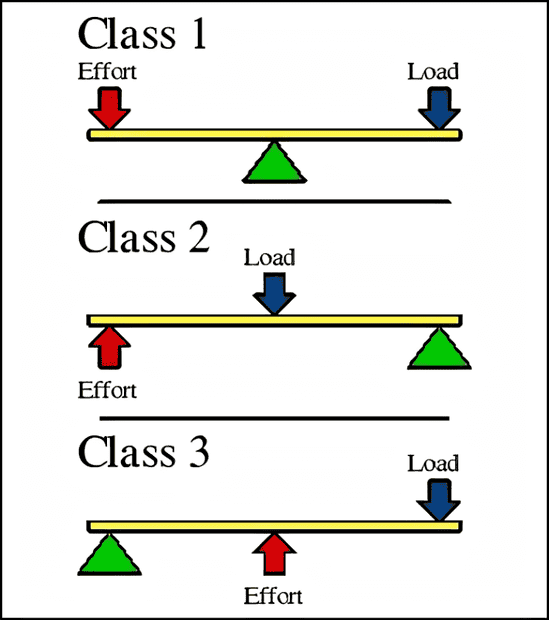# Analyzing a constraint eqn for a pulley system

guyvsdcsniper
Homework Statement:
Masses M1 and M2 are connected to a system of strings and pulleys
as shown. The strings are massless and inextensible, and the
pulleys are massless and frictionless. Find the acceleration of M1
Relevant Equations:
f=ma
I am having trouble creating the constraint equation for this pulley system.

I don't understand why the last 3 variables of the following constraint is divided by 2?

Could anyone help me understand why this is?Mentor
I don't understand why the last 3 variables of the following constraint is divided by 2?
That term represents the distance of the second pulley from ground level. (The entire expression is equivalent to saying that the height of the first pulley is fixed.)

•guyvsdcsniper
guyvsdcsniper
That term represents the distance of the second pulley from ground level. (The entire expression is equivalent to saying that the height of the first pulley is fixed.)
Oh I see. So since there are two strings connected to the second pulley, that distance is halved?

Mentor
So since there are two strings connected to the second pulley, that distance is halved?
Right.

•guyvsdcsniper
Homework Helper
Gold Member
...
I am having trouble creating the constraint equation for this pulley system.

I don't understand why the last 3 variables of the following constraint is divided by 2?

Could anyone help me understand why this is?
You can also analyze the system as a mechanism of instantaneous levers.
The anchored pulley works as a type 1 lever (mechanical advantage = 1).
The movil pulley works as a type 2 lever (mechanical advantage = 2 for M2 respect to M1, or 0.5 for M1 respect to M2).•guyvsdcsniper